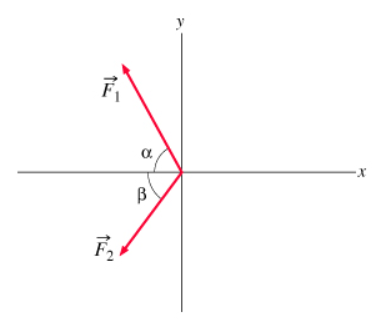# Problem: Two forces, F1 and F2, act at a point, as shown in the picture. (Fiqure 1) F1 has a magnitude of 8.80 N and is directed at an angle of α-65.0° above the negative x axis in the second quadrant. F2 has a magnitude of 5.40 N and is directed at an angle of β = 53.3° below the negative x axis in the third quadrant. A) What is the x component Fx of the resultant force?B) What is the y component Fy of the resultant force?C) What is the magnitude F of the resultant force?D) What is the angle ? that the resultant force forms with the negative x axis? In this problem, assume that positive angles are measured clockwise from the negative x axis.

###### FREE Expert Solution

Vector components:

2D vectors Magnitude:

$\overline{)\mathbf{|}\stackrel{\mathbf{⇀}}{\mathbit{A}}\mathbf{|}{\mathbf{=}}\sqrt{{{\mathbit{A}}_{\mathbit{x}}}^{\mathbf{2}}\mathbf{+}{{\mathbit{A}}_{\mathbit{y}}}^{\mathbf{2}}}}$

Direction:

$\overline{){\mathbf{tan}}{\mathbit{\theta }}{\mathbf{=}}\frac{{\mathbit{A}}_{\mathbit{y}}}{{\mathbit{A}}_{\mathbit{x}}}}$

90% (108 ratings)###### Problem Details

Two forces, F1 and F2, act at a point, as shown in the picture. (Fiqure 1) F1 has a magnitude of 8.80 N and is directed at an angle of α-65.0° above the negative x axis in the second quadrant. F2 has a magnitude of 5.40 N and is directed at an angle of β = 53.3° below the negative x axis in the third quadrant.A) What is the x component Fx of the resultant force?

B) What is the y component Fy of the resultant force?

C) What is the magnitude F of the resultant force?

D) What is the angle ? that the resultant force forms with the negative x axis? In this problem, assume that positive angles are measured clockwise from the negative x axis.